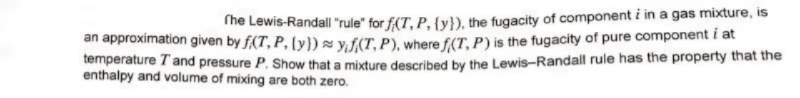# The Lewis-Randall "rule" for f(T, P, {y}), the fugacity of component i in a gas mixture, isan approximation given by f(T, P, ty)syf(T, P), where f(T, P) is the fugacity of pure component i attemperature T and pressure P. Show that a mixture described by the Lewis-Randall rule has the property that theenthalpy and volume of mixing are both zero.

Question

thermodynamicshelp_outlineImage TranscriptioncloseThe Lewis-Randall "rule" for f(T, P, {y}), the fugacity of component i in a gas mixture, is an approximation given by f(T, P, ty)syf(T, P), where f(T, P) is the fugacity of pure component i at temperature T and pressure P. Show that a mixture described by the Lewis-Randall rule has the property that the enthalpy and volume of mixing are both zero. fullscreen
check_circle

Step 1

Fugacity is used in case of real gas in place of pressure.

It denotes interaction between molecules of real gas.

It has same unit as of pressure.

Step 2

Lewis- Randall rule is applicable only for ideal solutions.

Lewis-Randall rule states that fugacity of component i in the ideal solution is directly proportional to its mole fraction which is shown below:

Where fi is proportionality constant

Step 3

The Lewis- Randall rule for the fugacity of component i in a gas  mixture is an approximation is giv...

### Want to see the full answer?

See Solution

#### Want to see this answer and more?

Solutions are written by subject experts who are available 24/7. Questions are typically answered within 1 hour.*

See Solution
*Response times may vary by subject and question.
Tagged in

### Thermodynamics